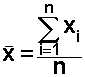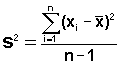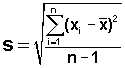## Calculator 4 - Statistics (Std Dev. Mean Variance)

###The following is a brief description of each of the statistical calculations performed by this calculator:

Mean: This is the arithmetic mean, commonly known as the average, and is defined by:where n is the sample size.

Sample Variance: This is essentially the average of the squared deviations from the mean, and is defined by:Standard Deviation: This is by far the most commonly used measure of variation.  It is given in the same units as the observations, and is the square root of the variance, as defined by:BASIC STATISTICAL CALCULATOR

Input Values

Input:

Database:

Running Statistics:

Sample Size:

Mean:

Sample Variance:

Standard Deviation:Example:

A series of hydraulic conductivities were calculated from slug tests conducted on six different monitoring wells within one contaminated property.  The conductivities were (in cm/s):

1.1 x 10-3; 1.4 x 10-4; 1.6 x10-3; 8.6 x 10-4; 6.7 x 10-4; 9.1 x 10-5

However, the result of 9.1 x 10-5 is thought to be from a well where silts have blocked the sand pack, causing the conductivity to appear lower than it should be.  What difference would it make to the mean, standard deviation and sample variation to dismiss this hydraulic conductivity from the data?

Solution:

 Without the data point With the data point sample size 5 6 mean 8.70E-04 7.40E-04 sample variance 2.90E-07 3.30E-07 standard deviation 5.40E-04 5.80E-04